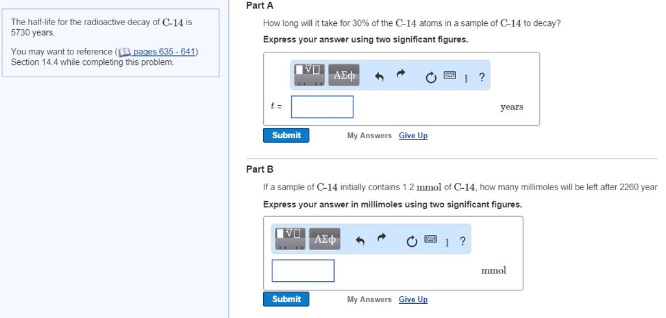# Problem: The half-life for the radioactive decay of C-14 is 5730 years. How long will it take for 30% of the C-14 atoms in a sample of C-14 to decay? Express your answer using two significant figures. If a sample of C-14 initially contains 1.2 mmol of C-14, how many millimoles will be left after 2260 year Express your answer in millimoles using two significant figures.

###### FREE Expert Solution
89% (140 ratings)###### Problem Details

The half-life for the radioactive decay of C-14 is 5730 years.

How long will it take for 30% of the C-14 atoms in a sample of C-14 to decay? Express your answer using two significant figures.

If a sample of C-14 initially contains 1.2 mmol of C-14, how many millimoles will be left after 2260 year Express your answer in millimoles using two significant figures.